# 1.基于Windows平台的Tensorflow安装

在Windows平台下进行Tensorflow的安装，需要打开Windows PowerShell，在我的计算机上安装的Tensorflow版本为1.1.0rc2，网址为 https://pypi.org/project/tensorflow/1.1.0rc2/，安装时在Windows PowerShell下面输入pip install tensorflow==1.1.0rc2，进行自动安装，当缺少相关组件时，进行相应的安装。安装成功后，如下图所示：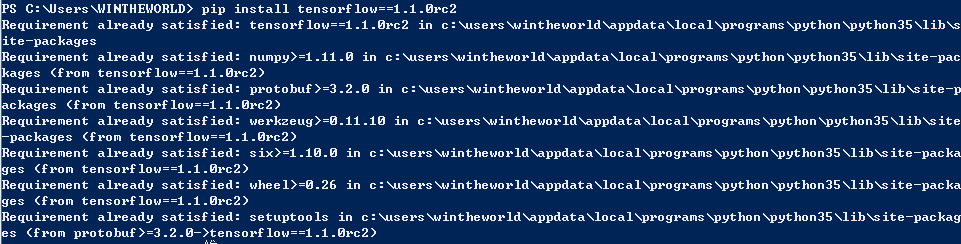此时可以对是否安装成功进行测试，在Windows PowerShell下输入Python，进行Python工作环境，输入import tensorflow，如果安装成功，此时将不会出现错误信息。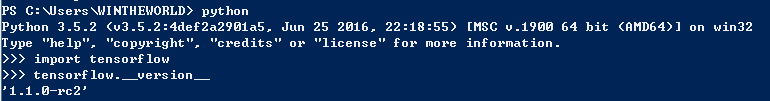# 通过寻找函数的权值和偏置，从而来学习Tensorflow。在tensorflow中定义变量要用tf.Variable()，因此在利用Tensorflow进行训练时的步骤为：

（1）定义损失函数；（2）定义优化器；（3）最小化损失函数；（4）初始化所有参数；（5）创建会话；（6）进行训练

完整代码如下所示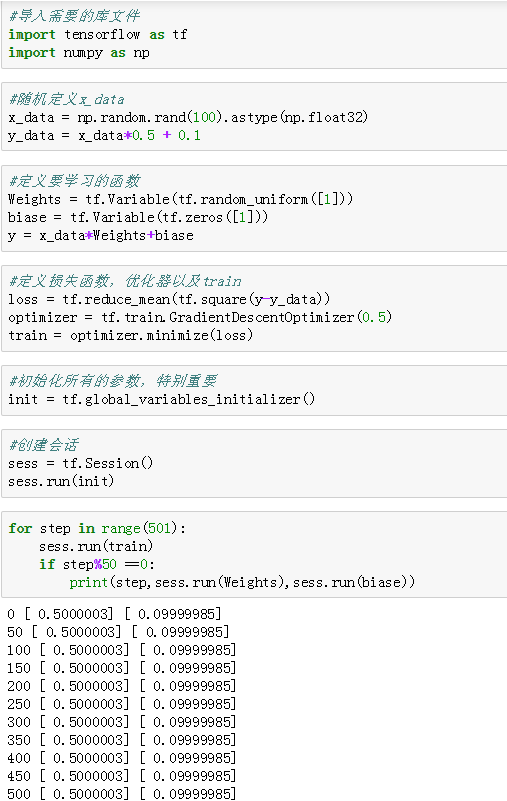# 在Tensorflow中，Session特别重要，Session是用来进行命令执行的语句。本次通过矩阵相乘的例子来解释Session的会话。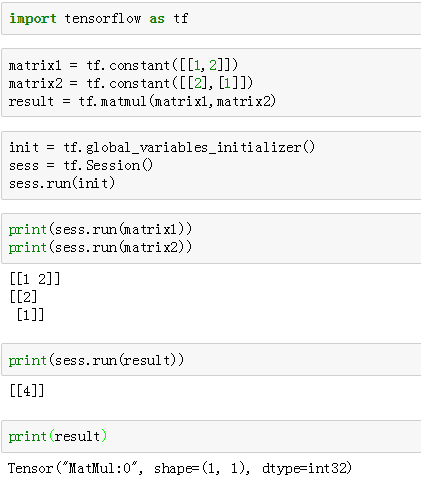# 4.Tensorflow Variable变量

在Tensorflow中，只有将变量定义为变量后，该变量才为变量，只有将常量定义为常量后，该常量才为常量，当在Tensorflow中定义了变量后，一定要进行初始化操作，并且对于变量的赋值，要利用tf.assign函数进行数值的叠加操作。具体代码如下所示。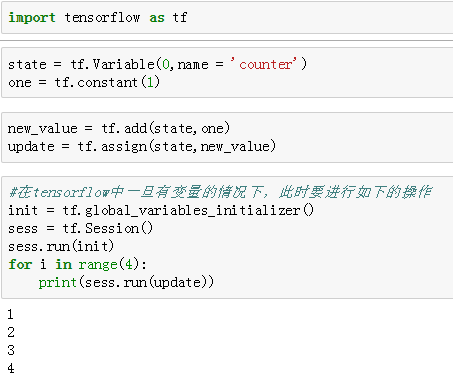# 5.Tensorflow placeholder

Tensorflow中的placeholder是变量的占位符，当利用了placeholder后，要在sess的run方法中，增加feed_dict字段，传入数值。具体的代码如下所示。

# 6.Tensorflow activation_function 激励函数

为了解决非线性问题，在Tensorflow中有如下的激励函数：Linear function，Step function，Ramp function，Softmax function等。其中relu为当输入小于0时，值为0，输入大于0时，为线性；dropout为了解决过拟合以及欠拟合问题；sigmoid常用于分类问题。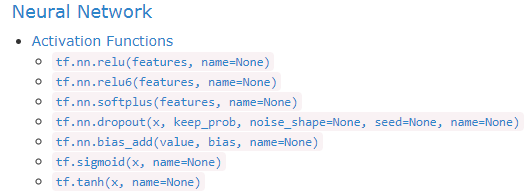# 7.Tensorflow 添加神经网络层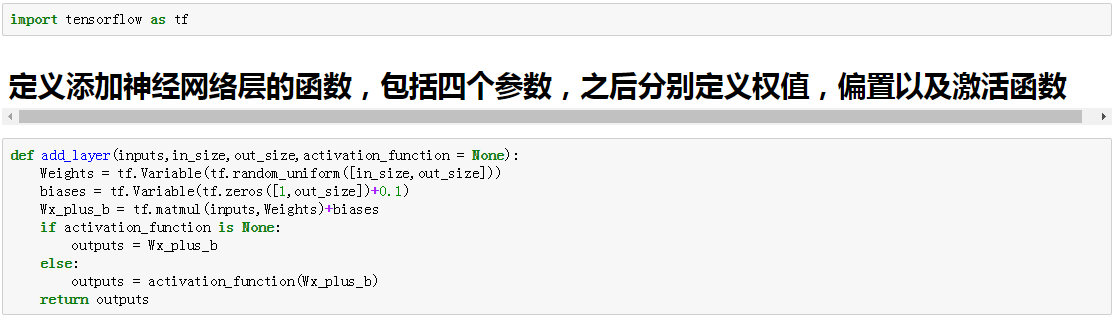# 8.Tensorflow 建立神经网络

1.数据类型不匹配

由于不同版本的Tensorflow会进行函数的更新，因此，会出现float64与float32数据格式不匹配的情况，此时要对数据进行格式转换，格式转换用numpy.float32()函数进行格式的转换。

2.训练后的损失值为NAN

这是由于loss计算后的值过小，同时选择的激活函数不适合，因此此时需要调整损失值的计算方式或者是更换激活函数或者是减小学习率，我这个是由于将tf.square()错打成了tf.sqrt()进行发现的这个问题。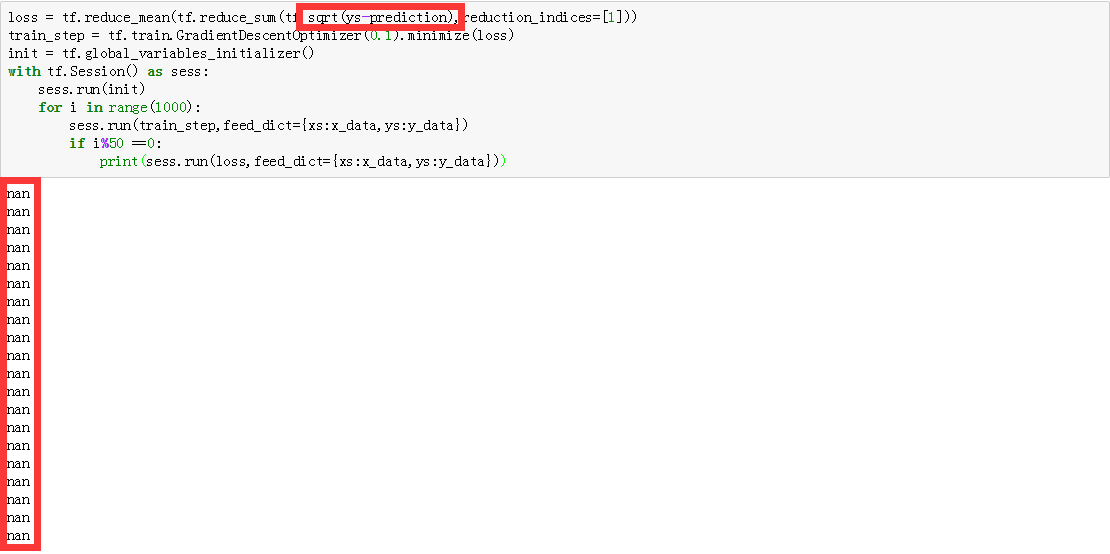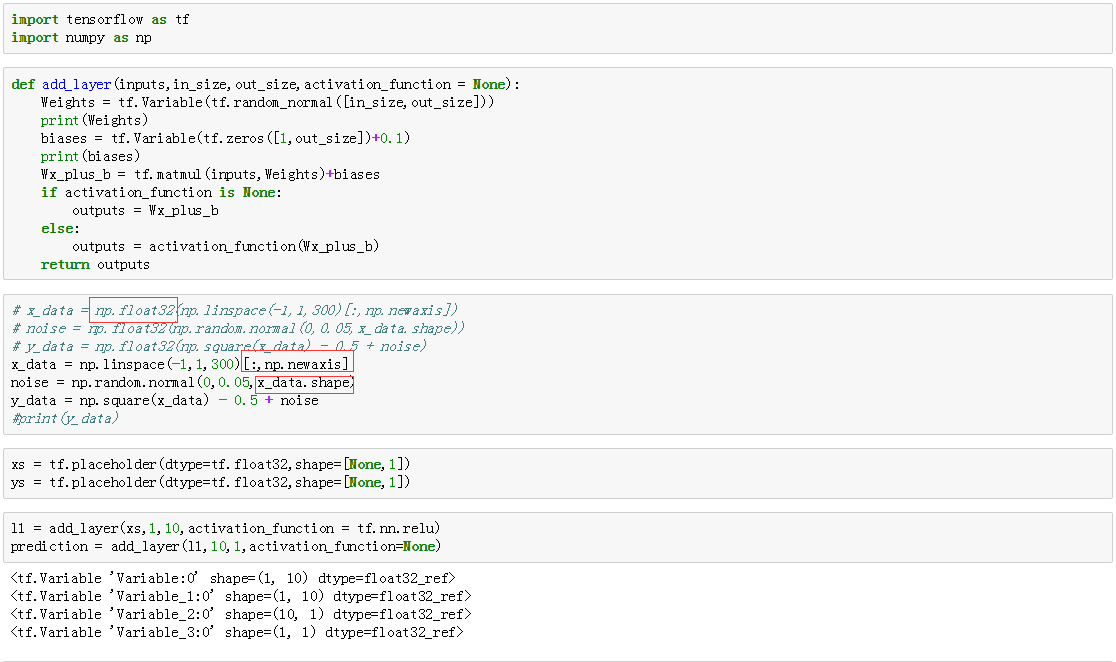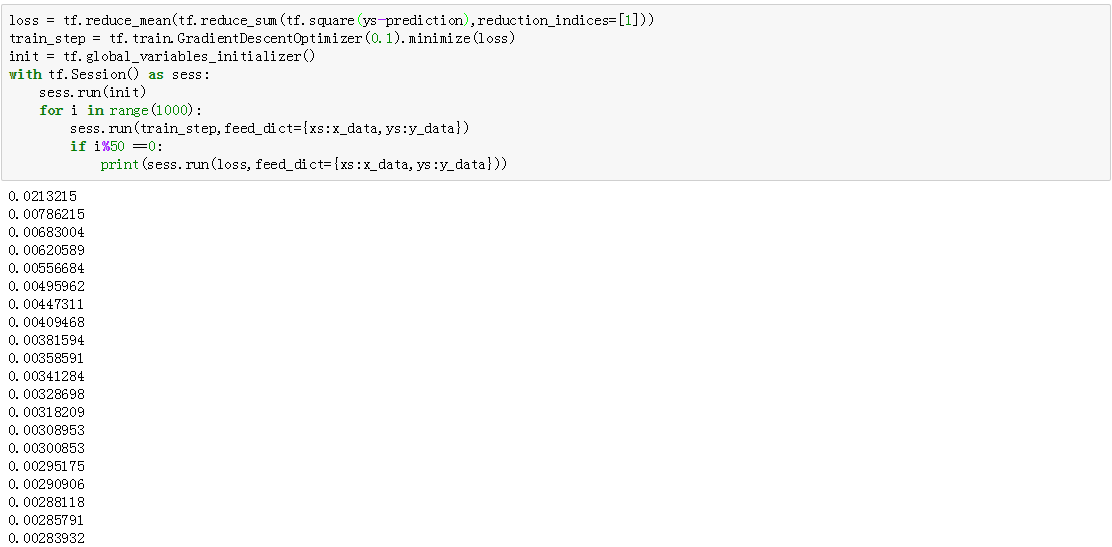# 9.Tensorflow结果显示

对于结果的显示，仅仅需要在8的所有代码里面加入下面方框中的语句就可以进行结果的显示，该结果的显示是动态进行显示的，为了能够进行动态的显示，使用plt.ion()函数，如果仅仅使用plt.show()函数，将仅仅会有静态的图像。
首先需要通过x_data预测出数据，通过ax.plot()将预测的数据与x_data画出来，参数‘r-’代表红色的连线，线段的宽度为5，通过plt.pause()进行时间的暂停。首先删除原有的线，之后增加新的线。由于开始时没有线，会报错，因此采用try捕捉错误，捕捉后不进行任何的操作。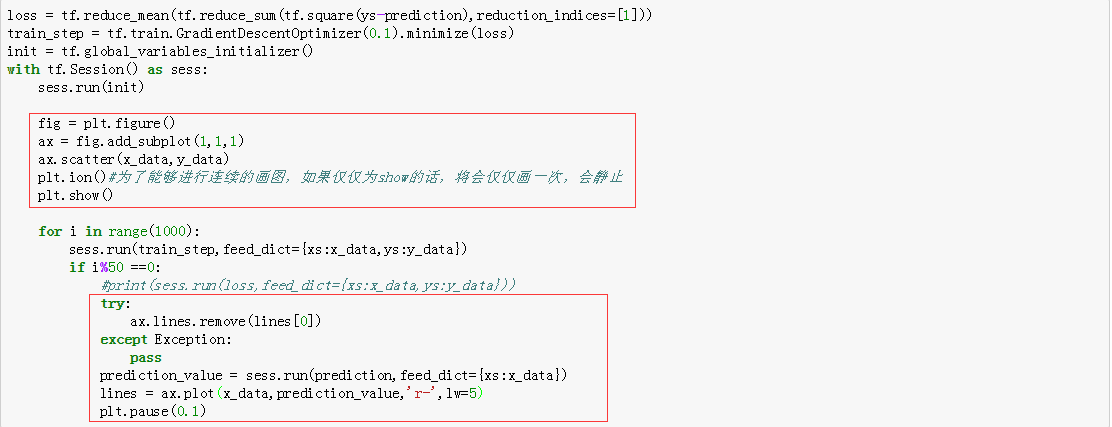注意，当在jupyter中进行如上操作时，会报错。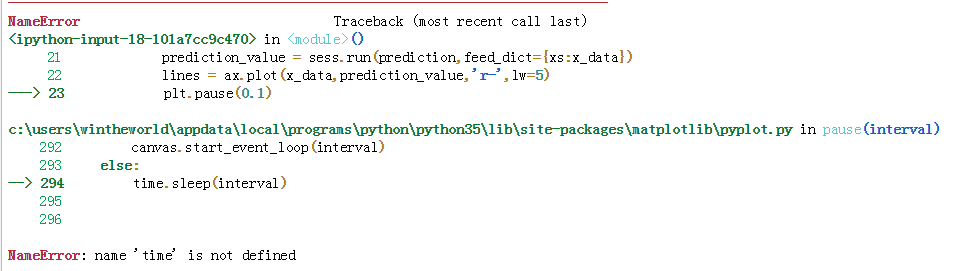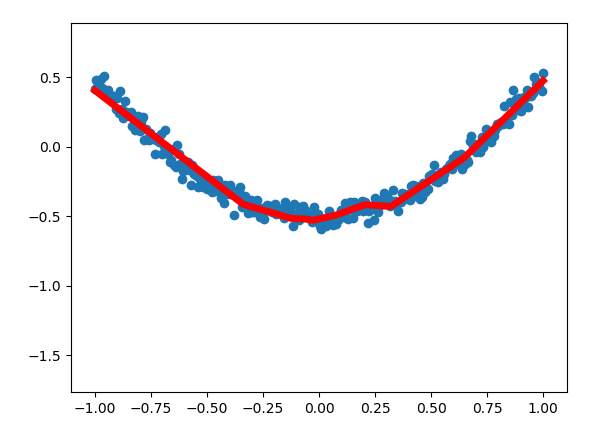# 11.可视化神经网络----使用Tensorboard可视化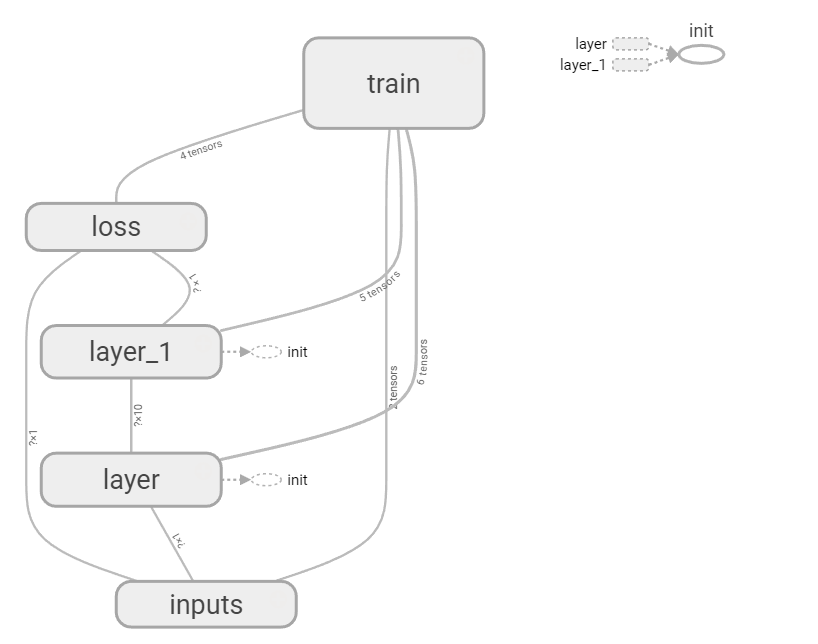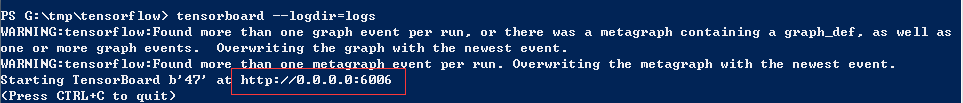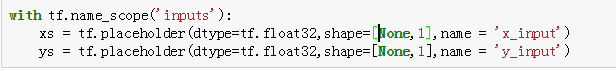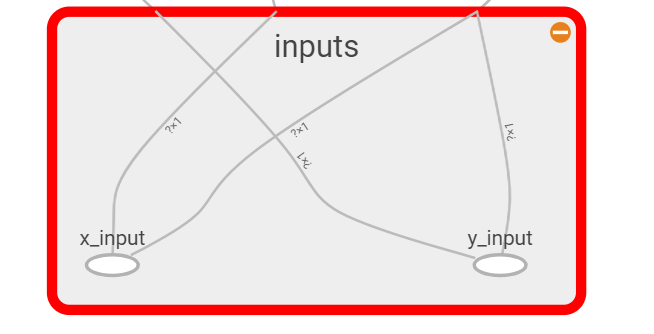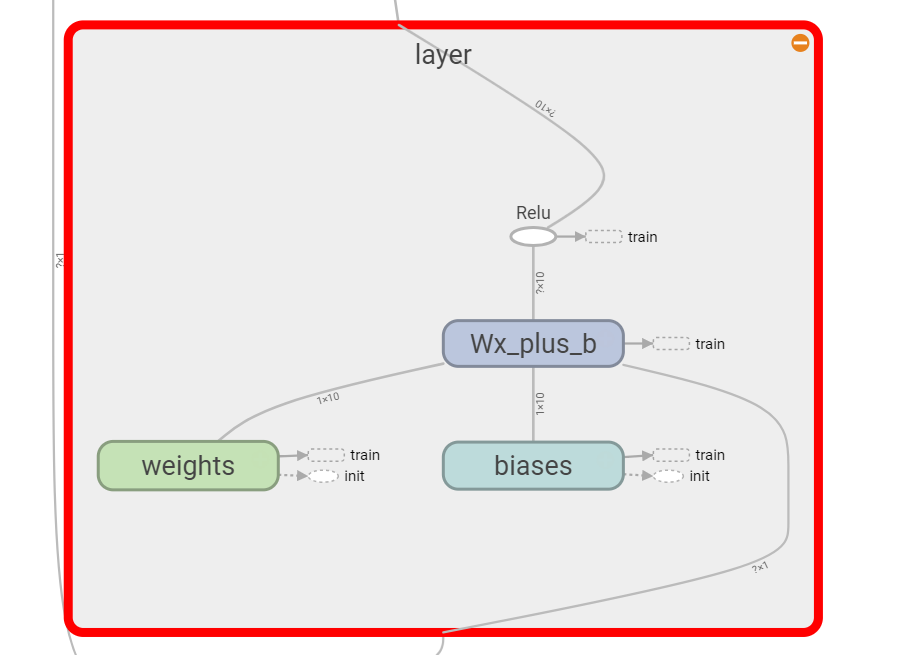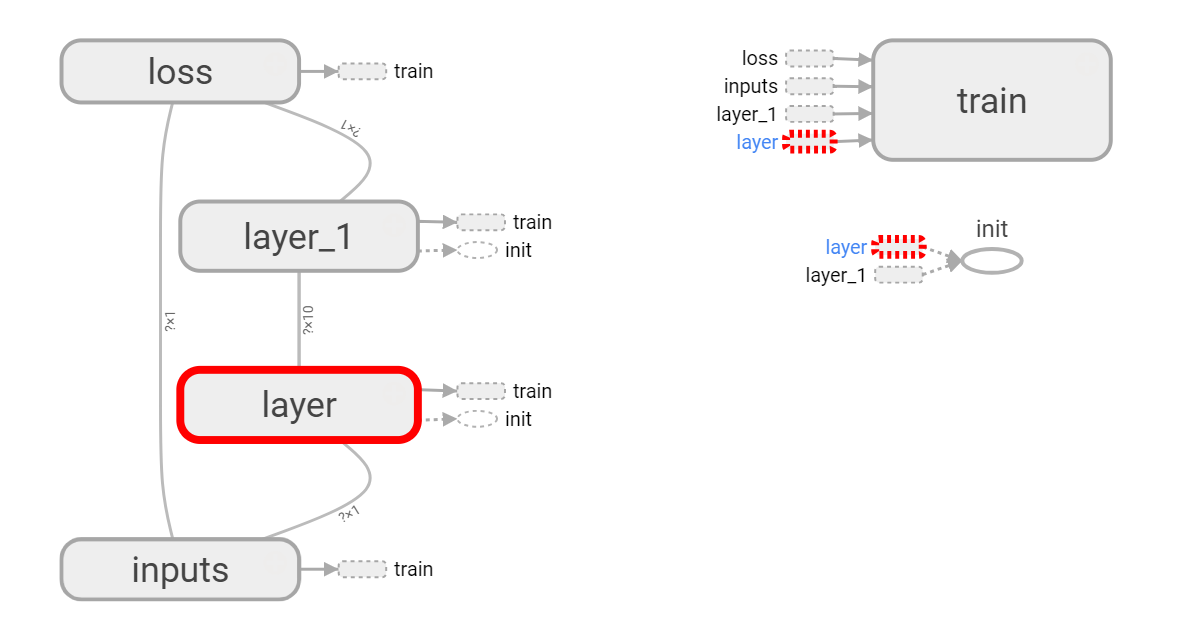# tf.merge_all_summaries()改变为tf.summary.merge_all()

tf.histogram_summary()改变为tf.summary.histogram()

tf.scalar_summary()改变为tf.summary.scalar()

tf.scalar_summary()改变为tf.summary.image()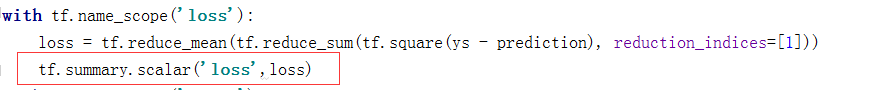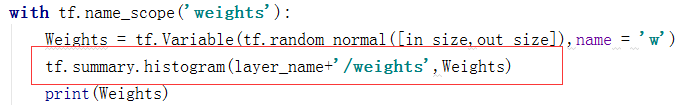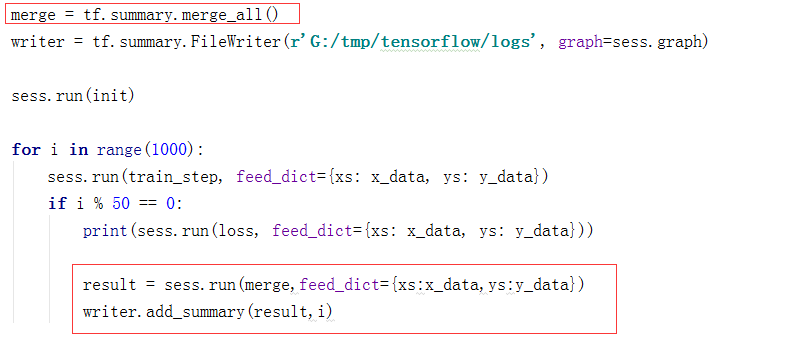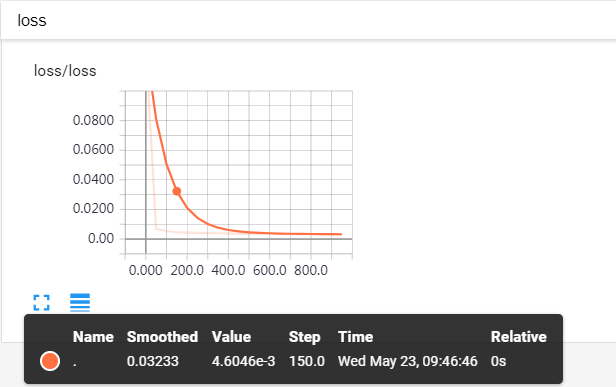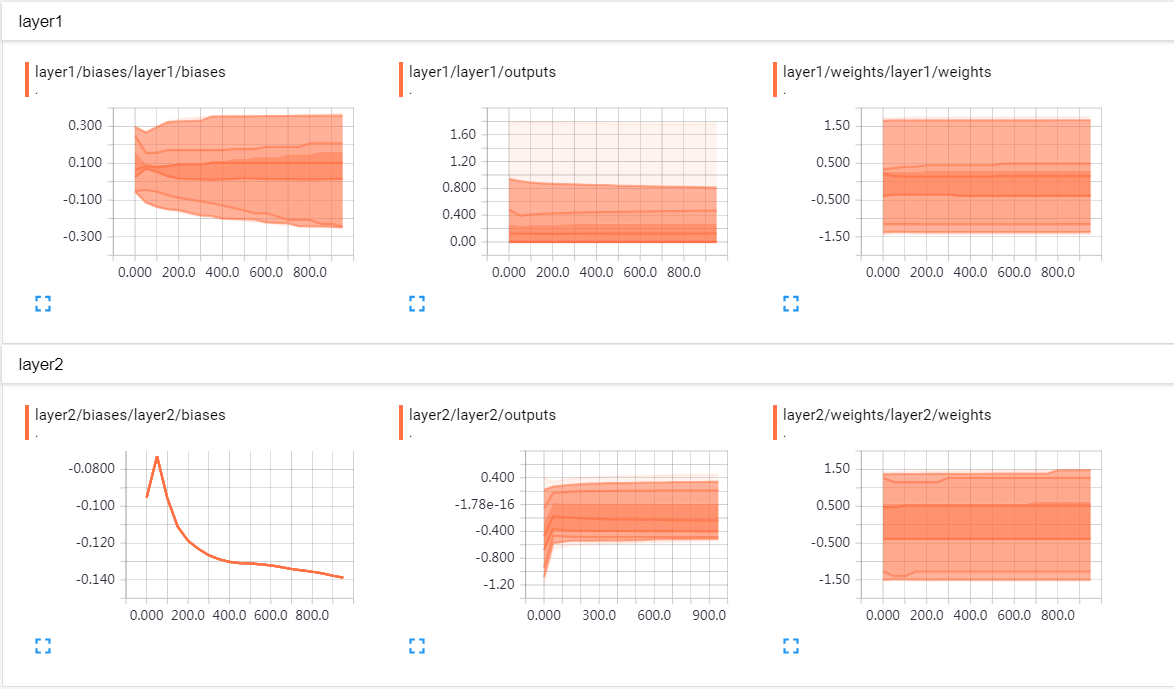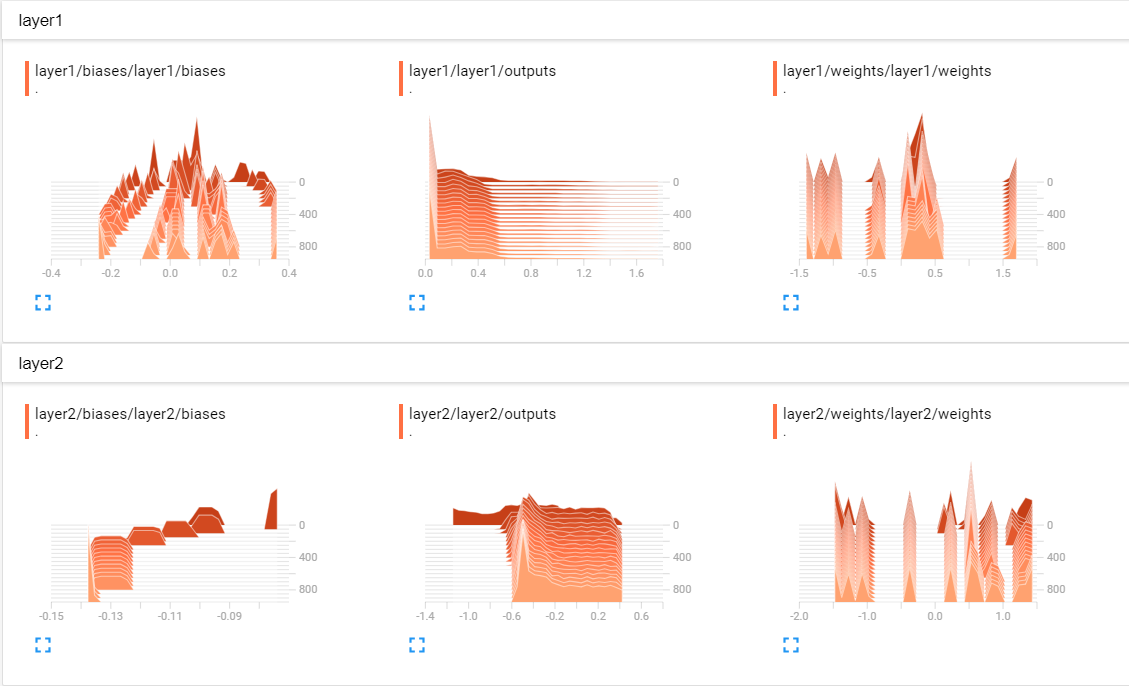（1）定义每一层：tf.name_scope(层的名字)

（2）定义每一层中变量的名字：在定义的变量后面加上name字段

（3）观察权值以及偏重的变化：tf.summary.histogram(随意名字，值)

（4）观察训练时的损失值：tf.summary.scalar(随意名字，值)

（5）将所有的值打包：merge = tf.summary.merge_all()

（6）写入本地文件：write = tf.summary.FileWriter(路径，graph = sess.graph)

（7）动态加载数据：result = sess.run(merge,feed_dict={xs:x_data,ys:y_data})

（8）写入文件：write.add_summary(result,i)

# 13.Tensorflow 分类学习

以上所有说的都跟回归相关，下面要利用Tensorflow进行分类学习，分类学习用的是很老的数据库，分类学习的Hello World，MNIST数据库。

代码如下：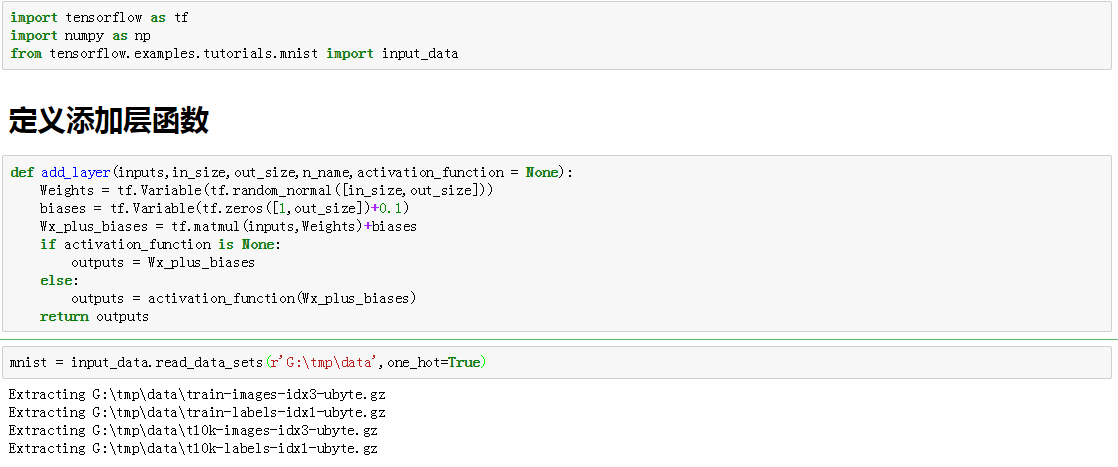需要在路径的位置换成自己的路径，如果没有数据库的话，将会进行下载。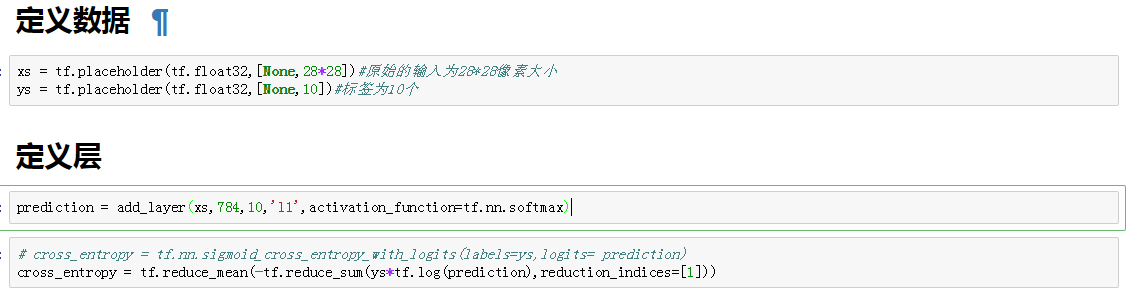定义了输入数据和输出数据，输入数据xs:28*28为原始数据中，每一个数据的大小，ys:10为采用one-hot编码的标签，本文使用softmax作为激励函数，激励函数输出的是概率值，最后定义损失函数。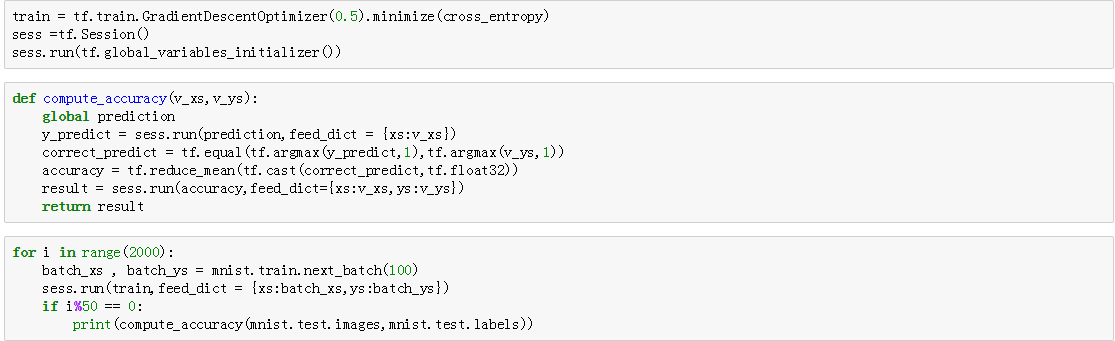# 14.Tensorflow 解决Overfitting问题

为了避免机器学习中，出现过拟合以及欠拟合问题，使用dropout来解决Overfitting问题。  没有进行dropout的结果如下所示：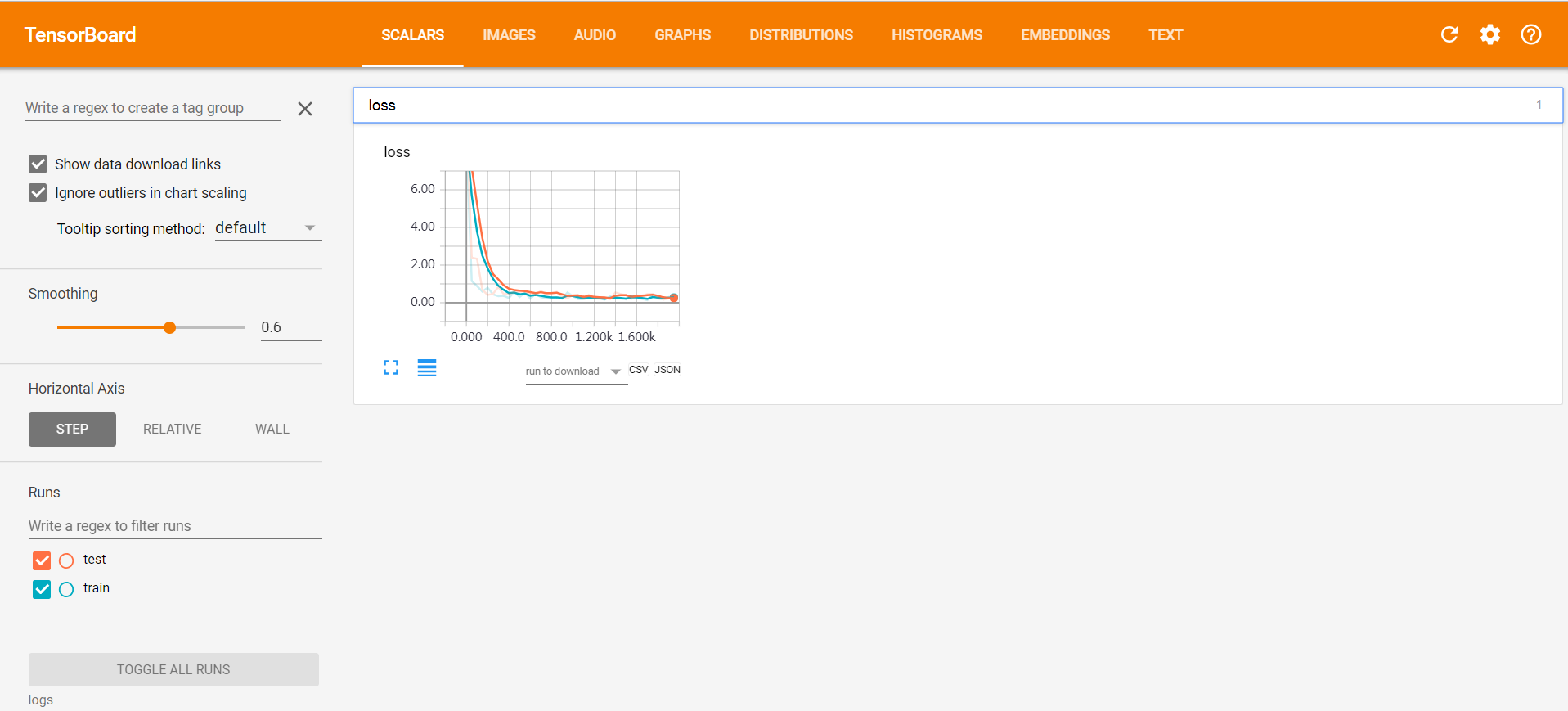加入了dropout之后的结果，dropout = 0.5: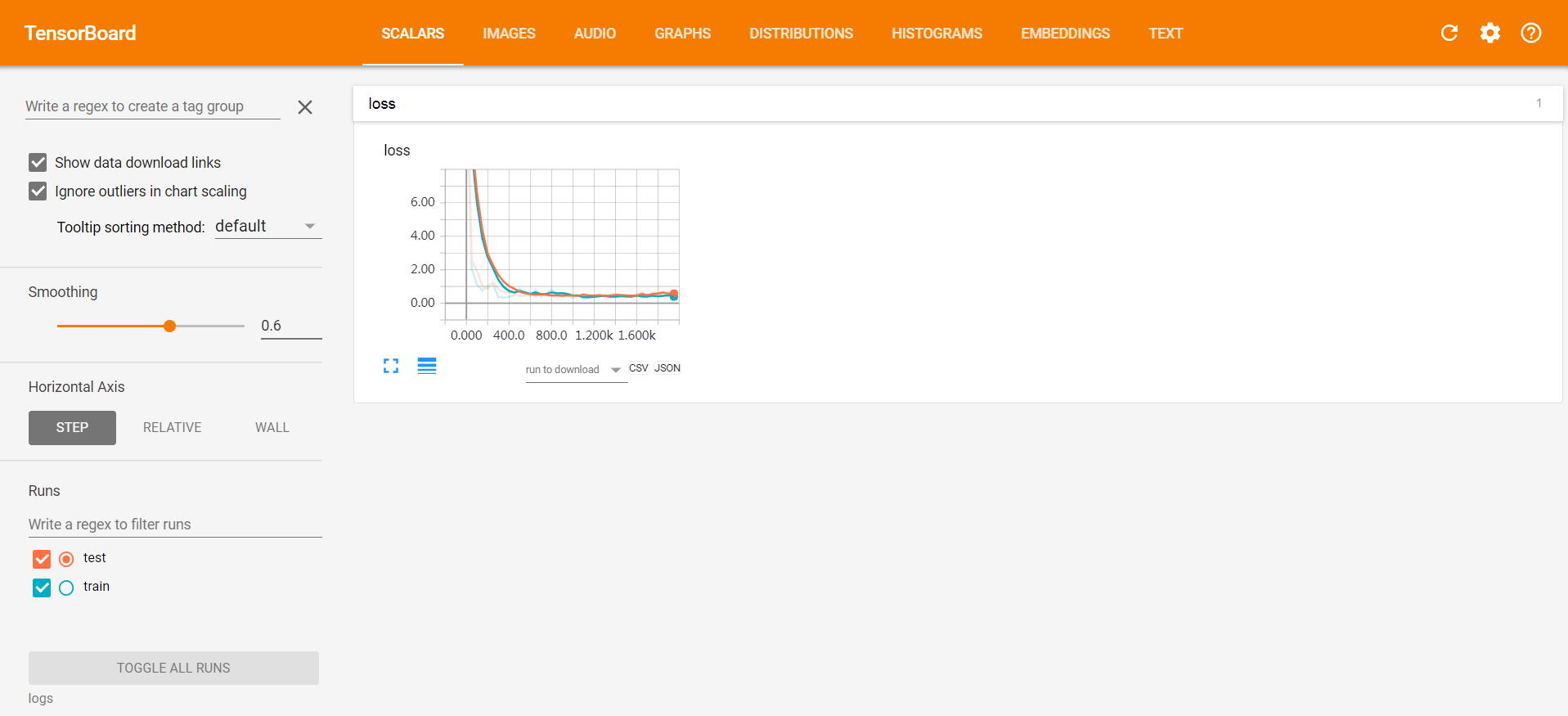在利用Tensorboard进行结果显示的时候，有的时候会出现图形很乱的情况，如下所示：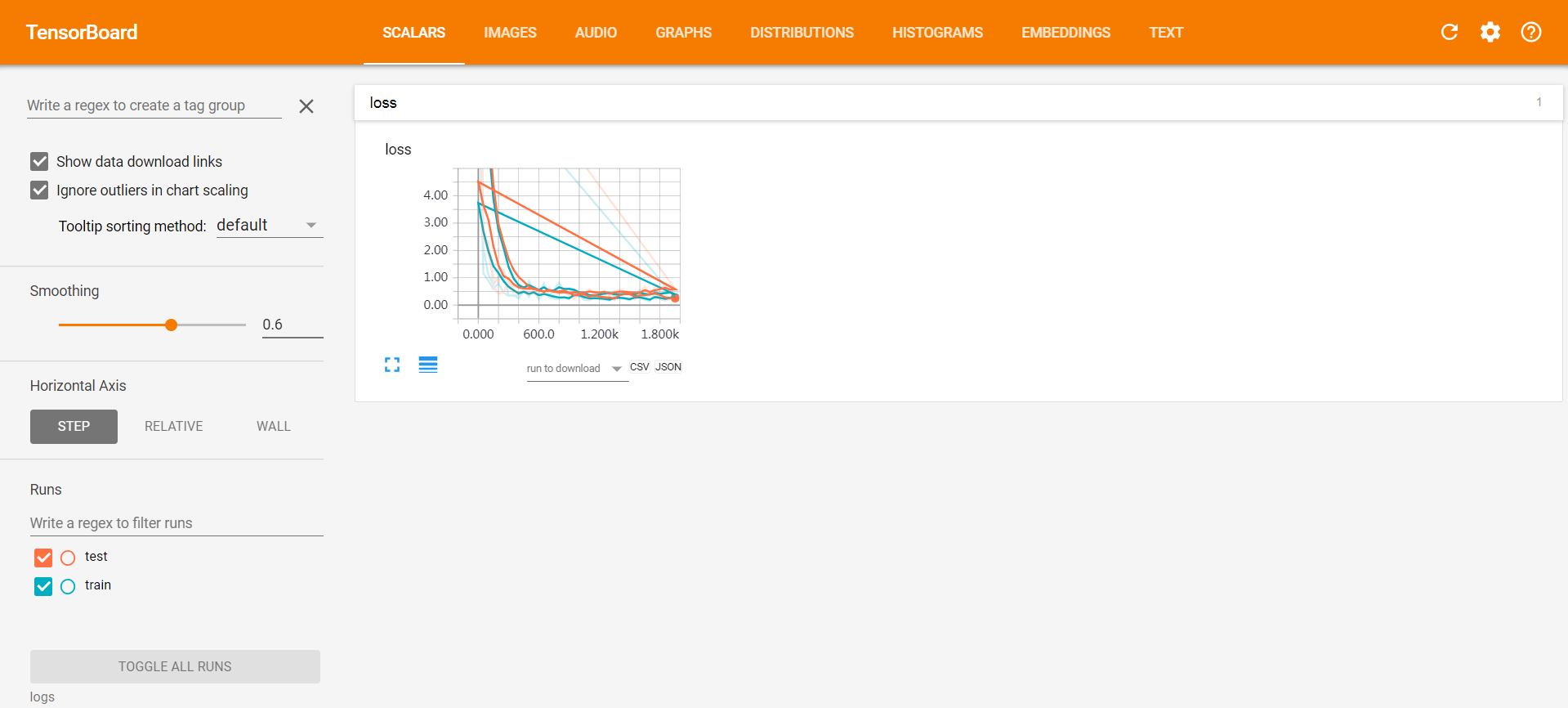这个时候，需要我们重新在Window PowerShell中重新输入tensorboard --logdir = logs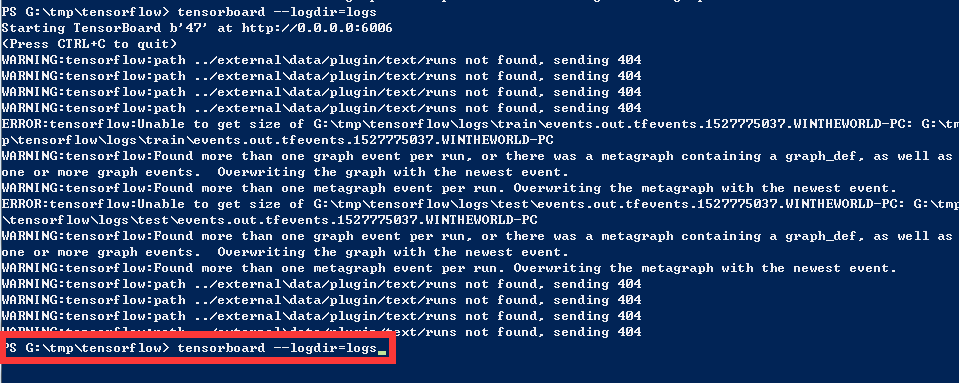同时将原有生成的日志文件清除干净，重新打开网址，则恢复正常。

# 15.Tensorflow CNN

通过神经网络对MNIST进行训练，最高只能达到88%左右，这样的正确率明显不能满足现实的需要，因此采用卷积神经网络的方式对MNIST进行测试。

在创建CNN时，需要进行权值的设定，偏置的设定，卷积层的设定，池化层的设定以及为了能够解决过拟合而进行的dropout，接下来分别对以上进行设定。

（1）权值的设定

def weightVariable(shape):
init = tf.random_normal(shape,stddev=0.01)
return tf.Variable(init)         

（2）偏置的设定

def biasVariable(shape):
init = tf.random_normal(shape)
return tf.Variable(init)

（3）卷积层的设定

def conv2d(x,W):
return tf.nn.conv2d(x,W,strides=[1,1,1,1],padding='SAME')

我们要注意的是，对于strides里面的参数设定，第一个参数一定是1，最后一个也一定是1，否则将会出现问题，即设定的形式为：[1,x_movement,y_movement,1]

（4）池化层的设定

def maxpool(x):
return tf.nn.max_pool(x,ksize=[1,2,2,1],strides=[1,2,2,1],padding='SAME')

我们要注意的是，对于maxpool里面的strides参数的设定，第一个以及最后一个参数一定是1，即设定形式为[1,x_movement,y_movement,1]，对于池化方式来说，有最大值池化方式以及平均值池化方式，同时对于padding来说也有两种padding的方式。

（5）dropout

def dropout(x,keep):
return tf.nn.dropout(x,keep)

dropout是为了克服过拟合问题，因此通过采用dropout的方式进行，通过选择一部分神经元设定为不工作的方式来进行参数的设定。

在进行了基本的参数功能设定后，搭建卷积神经网络：

def cnnLayer(classnum):
#第一层
W1 = weightVariable([3,3,3,32])#即卷积核为3*3大小，输入为3通道，输出为32通道
b1 = biasVariable()#对应32个输出通道
conv1 = tf.nn.relu(conv2d(x,W1)+b1)
pool1 = maxPool(conv1)
drop1 = dropout(pool1,keep_prob_5)
    #第二层
  W2 = weightVariable([3,3,32,64])#即卷积核为3*3大小，输入为32通道，输出为64通道
b2 = biasVariable()#对应64个输出通道
conv2 = tf.nn.relu(conv2d(drop1,W2)+b2)
pool2 = maxPool(conv2)
drop2 = dropout(pool2,keep_prob_5)
  #第三层
W3 = weightVariable([3,3,64,64])#即卷积核为3*3大小，输入为64通道，输出为64通道
b3 = biasVariable()#对应64个输出通道
conv3 = tf.nn.relu(conv2d(drop2,W3)+b3)
pool3 = maxPool(conv3)
drop3 = dropout(pool3,keep_prob_75)
在我们的程序中，需要对输入神经元与输出神经元的神经网络节点进行设置，输入图片为64*64大小的彩色图像，因此input = 64*64*3，将输入的图片进过第
一层神经网络，设置第一层网络的卷积核大小为3*3，由于是彩色图像，因此图像的通道数为RGB，3通道，如果是灰度图像，此时的输入将为1通道的灰度图像，设
置输出为32，即32个特征，之后将提取后的特征进行线性相加后进行非线性映射，之后对非线性映射后的结果，进行池化操作，池化操作的模板为2*2，进行最大值
池化，为了防止过拟合，使用dropout的方式，最后经过以上的步骤后，图像变为了32*32*32；将32*32*32规模大小的图像输入第二层神经网络，同样经过以上的步
骤，最后输出图像的规模大小为16*16*64，将第二层输出的图像输入到第三层神经网络，第三层神经网络的输入图片大小为16*16*64，经过同样的处理方式，结果
为8*8*64，经过三次神经网络的处理，由原始的64*64*3的图像变为了8*8*64大小规模的图像。接下来，我们将8*8*64大小的图像输入到全连接层，全连接层的输

入神经元个数为8*8*64，即4096个神经元，全连接神经元的输出为512个神经元，将512神经元网络进行reshape，成为1行512列的数据，之后输入分类层，分类层
的输入为512，输入为将要分类的类别数。
    #全连接层,最后将drop3的结果输入到全连接层，在全连接层后面最后加入一个分类的层 
   Wf = weightVariable([8*8*64,512])
    bf = biasVariable()
drop3_flat = tf.reshape(drop3,[-1,8*8*64])
dense = tf.nn.relu(tf.matmul(drop3_flat,Wf)+bf)
dropf = dropout(dense,keep_prob_75)
    #输出层
Wout = weightVariable([512,classnum])
bout = biasVariable([classnum])
out = tf.add(tf.matmul(dropf,Wout),bout)
return out

对于上面红色字体标明的地方特别的重要，要仔细的进行理解。

本文使用的网络模型为：input->conv1->maxpool1->conv2->maxpool2->conv3->maxpool3->全连接层->输出层

对于每一层图像规模大小为：

 网络名称 卷积模板（padding = ‘SAME’） 图像规模 input 64*64*3 conv1 3*3 64*64*32 maxpool1 2*2 32*32*32 conv2 3*3 32*32*64 maxpool2 2*2 16*16*64 conv3 3*3 16*16*64 maxpool3 2*2 8*8*64 全连接层 reshape(4096) 分类层 类别个数

利用以上的网络结构，进行数据的分类。

在第13节，我们已经定义了传统的神经网络进行MNIST的识别，通过结果我们可以发现，最终的正确率仅仅能够达到88%左右，通过将传统的神经网络进行替换，替换成我们的CNN模型，利用CNN模型进行训练以及识别，我们可以发现，正确率明显高于浅层神经网络。为了防止过拟合问题，在每一层神经网络增加dropout，其中卷积层dropout = 0.5，全连接层dropout = 0.75。

与第13的程序相比，程序变动的地方很少，为了能够将原始数据输入到神经网络中，需要将原始数据进行转换：

    xs = tf.placeholder(tf.float32,[None,28*28])
之后对数据做如下处理
x_image = tf.reshape(xs,[-1,28,28,1])

由于增加了dropout，因此需要添加相应的代码如下：

    keep_prob_5 = tf.placeholder(float32)
keep_prob_75 = tf.placeholder(float32)
最后调用神经网络结构：
    prediction = cnnLayer(xs_image,keep_prob_5,keep_prob_75,classnum)

由于增加了dropout，因此需要在训练的feed_dict中增加相应的属性参数，设置的属性参数如下：

    sess.run(train,feed_dict={xs:batch_xs,ys:batch_ys,keep_prob_5:0.5,keep_prob_75:0.75})

对于其他的均不用更换。

在实际的应用中，也许会出现最终的识别正确率并不改变，并且很小，这个时候，我们要对我们的网络进行调节，在我的实践中，发现正确率不变，由于没有在最后的分类层添加上非线性的约束，即

tf.nn.Softmax(tf.add(tf.matmul(dropf,Wout),bout))

最终的结果如下所示：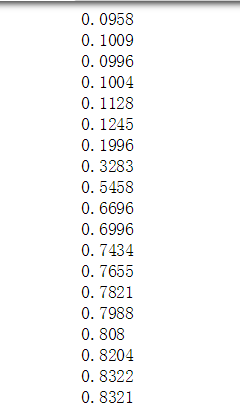->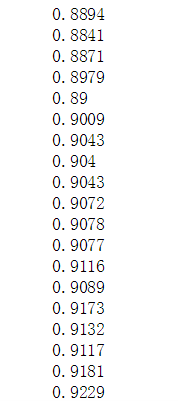->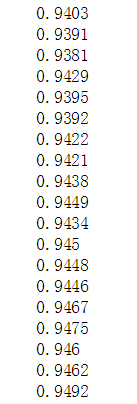->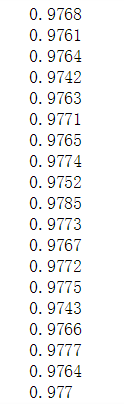通过结果可以发现，当准确率达到97.7%之后，结果正确率上升将会更加的困难，因此最后正确率可以达到97%以上。

# 16.Tensorflow 模型的保存以及读取

在利用数据进行训练后，生成模型，对模型进行保存，模型文件如下所示：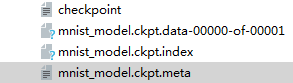第一个文件为保存点文件，第三个文件为模型的数据文件，保存训练后的参数，第四个文件为保存的图结构。为了对模型文件进行调用，使用如下的函数进行调用：

首先要恢复网络的图结构

saver = tf.train.import_meta_graph('./mnist_model.ckpt.meta')

接下来将训练后的参数进行加载

saver.restore(sess,'./mnist_model.ckpt')

这样就可以进行模型的调用，注意，在进行模型的加载时，需要重新加载网络的模型，对于本网络来说，网络的训练代码如下所示：

import tensorflow as tf
from tensorflow.examples.tutorials.mnist import input_data

mnist = input_data.read_data_sets(r'G:\tmp\data',one_hot=True)

xs = tf.placeholder(tf.float32,[None,28*28])#原始的输入为28*28像素大小
ys = tf.placeholder(tf.float32,[None,10])#标签为10个
xs_image = tf.reshape(xs,[-1,28,28,1])
keep_prob_5 = tf.placeholder(tf.float32)
keep_prob_75 = tf.placeholder(tf.float32)

def weightVariable(shape,name):
init = tf.random_normal(shape,stddev=0.01)
return tf.Variable(init,name)

def biasVariable(shape,name):
init = tf.constant(0.1,shape=shape)
return tf.Variable(init,name)

def conv2d(x,W):
return tf.nn.conv2d(x,W,strides=[1,1,1,1],padding='SAME')

def maxpool(x):
return tf.nn.max_pool(x,ksize=[1,2,2,1],strides=[1,2,2,1],padding='SAME')

def dropout(x,keep):
return tf.nn.dropout(x,keep)

def cnnLayer(xs_image,keep_prob_5,keep_prob_75,classnum):
#第一层
W1 = weightVariable([5,5,1,32],name='W1')#原始图像是灰度图像
b1 = biasVariable(,name='b1')
conv1 = tf.nn.relu(conv2d(xs_image,W1)+b1)
pool1 = maxpool(conv1)
drop1 = dropout(pool1,keep_prob_5) #经过处理后结果为14*14*32
#第二层
W2 = weightVariable([5,5,32,64],name='W2')
b2 = biasVariable(,name='b2')
conv2 = tf.nn.relu(conv2d(drop1,W2)+b2)
pool2 = maxpool(conv2)
drop2 = dropout(pool2,keep_prob_5) #经过处理后结果为7*7*64
#全连接层
Wf = weightVariable([7*7*64,1024],name='Wf')
bf = biasVariable(,name='bf')
drop2_flat = tf.reshape(drop2,[-1,7*7*64])
dense = tf.nn.relu(tf.matmul(drop2_flat,Wf)+bf)
dropf = dropout(dense,keep_prob_75)#经过处理后结果为1*512
#分类层
Wout = weightVariable([1024,classnum],name='Wout')
bout = biasVariable([classnum],name='bout')
out = tf.nn.softmax(tf.add(tf.matmul(dropf,Wout),bout))
return out

prediction = cnnLayer(xs_image,0.5,0.75,10)

cross_entropy = tf.reduce_mean(-tf.reduce_sum(ys*tf.log(prediction),reduction_indices=))

train = tf.train.AdamOptimizer(1e-4).minimize(cross_entropy)
sess =tf.Session()
sess.run(tf.global_variables_initializer())

saver = tf.train.Saver()

def compute_accuracy(v_xs,v_ys):
global prediction
y_predict = sess.run(prediction,feed_dict = {xs:v_xs,keep_prob_5:1.0,keep_prob_75:1.0})
correct_predict = tf.equal(tf.argmax(y_predict,1),tf.argmax(v_ys,1))
accuracy = tf.reduce_mean(tf.cast(correct_predict,tf.float32))
result = sess.run(accuracy,feed_dict={xs:v_xs,ys:v_ys,keep_prob_5:1.0,keep_prob_75:1.0})
return result

for i in range(4000):
batch_xs , batch_ys = mnist.train.next_batch(100)
sess.run(train,feed_dict = {xs:batch_xs,ys:batch_ys,keep_prob_5:0.5,keep_prob_75:0.75})
if i%50 == 0:
print(compute_accuracy(mnist.test.images,mnist.test.labels))

save_path = saver.save(sess,'./mnist_model.ckpt')

加载训练后的模型进行预测的代码如下所示：

import tensorflow as tf
import numpy as np
from PIL import Image

xs = tf.placeholder(tf.float32,[None,28*28])#原始的输入为28*28像素大小
xs_image = tf.reshape(xs,[-1,28,28,1])
keep_prob_5 = tf.placeholder(tf.float32)
keep_prob_75 = tf.placeholder(tf.float32)

def weightVariable(shape,name):
init = tf.random_normal(shape,stddev=0.01)
return tf.Variable(init,name)

def biasVariable(shape,name):
init = tf.random_normal(shape)
return tf.Variable(init,name)

def conv2d(x,W):
return tf.nn.conv2d(x,W,strides=[1,1,1,1],padding='SAME')

def maxpool(x):
return tf.nn.max_pool(x,ksize=[1,2,2,1],strides=[1,2,2,1],padding='SAME')

def dropout(x,keep):
return tf.nn.dropout(x,keep)

def cnnLayer(xs_image,keep_prob_5,keep_prob_75,classnum):
#第一层
W1 = weightVariable([5,5,1,32],name='W1')#原始图像是灰度图像
b1 = biasVariable(,name='b1')
conv1 = tf.nn.relu(conv2d(xs_image,W1)+b1)
pool1 = maxpool(conv1)
drop1 = dropout(pool1,keep_prob_5) #经过处理后结果为14*14*32
#第二层
W2 = weightVariable([5,5,32,64],name='W2')
b2 = biasVariable(,name='b2')
conv2 = tf.nn.relu(conv2d(drop1,W2)+b2)
pool2 = maxpool(conv2)
drop2 = dropout(pool2,keep_prob_5) #经过处理后结果为7*7*64
#全连接层
Wf = weightVariable([7*7*64,1024],name='Wf')
bf = biasVariable(,name='bf')
drop2_flat = tf.reshape(drop2,[-1,7*7*64])
dense = tf.nn.relu(tf.matmul(drop2_flat,Wf)+bf)
dropf = dropout(dense,keep_prob_75)#经过处理后结果为1*512
#分类层
Wout = weightVariable([1024,classnum],name='Wout')
bout = biasVariable([classnum],name='bout')
out = tf.nn.softmax(tf.add(tf.matmul(dropf,Wout),bout))
return out

cnnLayer = cnnLayer(xs_image,1,1,10)

init_op = tf.global_variables_initializer()

saver = tf.train.import_meta_graph('./mnist_model.ckpt.meta')
with tf.Session() as sess:
sess.run(init_op)
saver.restore(sess,'./mnist_model.ckpt')

image_path = r'G:\tmp\picturedata\originalPicture\14.bmp'
img = Image.open(image_path).convert('L')
image_shape = np.reshape(img,28*28)
real_x = np.array([1-image_shape])
y = sess.run(cnnLayer,feed_dict={xs:real_x,keep_prob_5:1,keep_prob_75:1})
print(np.argmax(y))


注意观察这两个代码，将会将相同的部分标红，也就是重新在预测网络时重新加载的网络结构。最终的结果如下所示：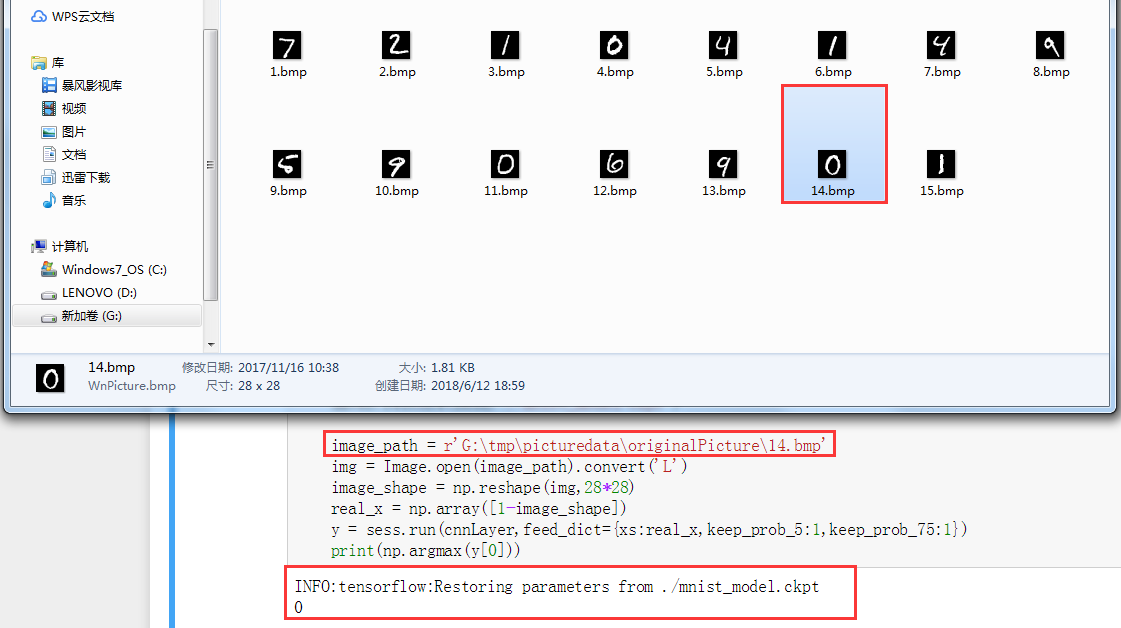在实际的测试中，我发现了一个问题，就是不知道如何设置dropout的数值，以及在什么地方设置dropout的数值，并且由于训练的轮数较少，因此识别的正确率不高，并且对于同一图像，识别的结果也不完全相同，但是我发现，在进行模型的训练时，正确率却很高，这是一个还没有解决的问题。至少应该可以知道如何设置dropout以及在什么地方设置dropout，并且识别的准确率提高，即使识别的准确率不高，对于同一副图像也应该是相同的识别结果，而不应该总是进行变化，但是本节仅仅是学习如何进行模型的调用。

为了能够更加清晰地说明模型的保存以及模型的调用，使用如下的例子进行讲解：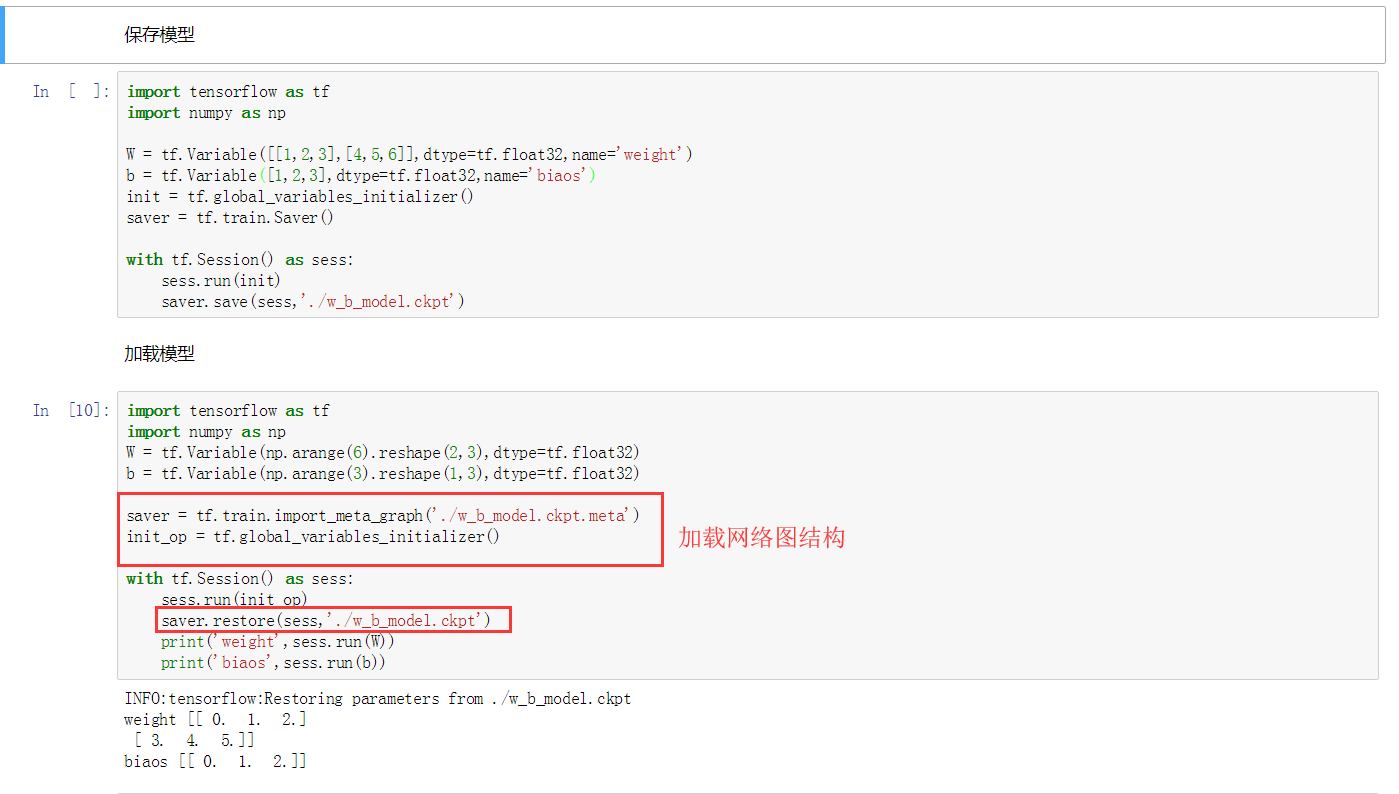对于当前版本的模型调用，需要加载网络的图结构，加载完成网络的图结构后，加载训练后的数据，便可以很好的进行模型的加载。

# 17.Tensorflow RNN 循环神经网络

卷积神经网络告一段落，对于网络的熟练应用，就是要能够多次使用，接下来，将要学习RNN即循环神经网络。

# 18.Tensorflow Autoencoder

Autoencoder是一种非监督的学习方式。未完待续

未完待续

# 20.Tensorflow Batch normalization批标准化

未完待续

在学习了Tensorflow基础知识后，将会利用Tensorflow实现一些应用，以及算法的实现。